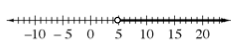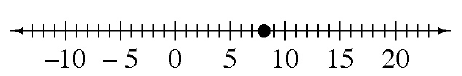### Home > CC1MN > Chapter 9 Unit 9 > Lesson CC1: 9.2.3 > Problem9-65

9-65.

Solve each of the following equations or inequalities and graph the solution on a number line.

1. $3x \gt 14$

Make the inequality an equation and use inverse operations to undo the equation and solve for the variable.

$3\textit{x} = 14$

$\frac{3\textit{x}}{3} = \frac{14}{3}$

$\textit{x} = 4\frac{2}{3}$

Make a number line and use a dot to represent the solution of the equation. Then, test numbers on both sides of the solution to see which side makes the inequality true and graph the rest of the solution.$\text{Notice that the circle is open because the inequality includes all}$

$\text{numbers from }4\frac{2}{3}\text{ and up but does not actually include }4\frac{2}{3}\text{ itself.}$

2. $8y = 64.72$

Try undoing the equation using inverse operations.

y = 64.72 ÷ 8
y = 8.09

Now use a dot to represent the solution on a number line.3. $6w ≤ 48.618$

See part (a).